1) State whether true or false. Correct the statement if they are false :
(a) Every negative integer is larger than positive integer.
(b) – 6 lies to the left of −5.
(c) Zero is neither negative integer nor a positive integer.
(d) 500 m below the sea level would be represented as a positive
integer.
(e) Further a number from zero on the left, smaller is its value.
(f) The value of the number decreases as we move towards right on
the number line.
Ans: (a) False. Every negative integer is smaller than positive integer.
(b) True.
(c) True.
(d) False. It would be represented as a negative integer.
(e) True.
(f) False. The value of the number increases as we move to the right on the number line.

2) Write down the numbers between :
(a) −7 and −1
(b) 5 and −4
(c) 0 and 6
(d) −9 and 3
Ans: (a) −6, −5, −4, −3, −2
(b) 4, 3, 2, 1, 0, −1, −2, −3
(c) 1, 2, 3, 4, 5
(d) −8, −7, −6, −5, −4, −3,−-2, −1 ,1, 2

3) Write the following numbers with appropriate sign :
(a) 30˚ C below 0˚ C temperature.
(b) 150 m below sea level.
(c) 115˚ C above 0˚ C temperature.
(d) Loss of ₹ 6500.
(e) Profit of ₹ 12500.
Ans: (a) – 30 .
(b) – 150 .
(c) +115 .
(d) – 6500 .
(e) + 12500 .

4) Represent − 2, 6, −8, 1, and −3 on the number line.
Ans: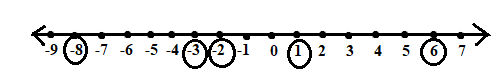5) Complete the following pairs of numbers using :
(a) 0 ___˃____ −8
(b) – 22 ___˃____ − 37
(c) – 17 ˂____ 17
(d) 1 ___˃____ 0

(e) (− 4) + (−11) ˂ (− 4) – (−11)
− 4 − 11 ˂ − 4 + 11
− 15 ˂ 7
(f) −23 + (−9) ≤ 23 – (− 9)

− 23 – 9 ≤ 23 +9
−32  ≤ 32
(g) – 47 – (− 13) = − 21 – 13

− 47 + 13 = − 34
− 34 = −34

6) Find the following :
(1) +7 + (− 13)
(2) (− 23) + (+10)
(3) (+ 20) + (− 17)
(4) (− 117) + (− 82)
(5) – 42 – 6 + (− 60)
(6) 102 – (− 83) + (− 35)
(7) (−14) – (−7) + 22
Ans :
(1) + 7 – 13 = − 6

(2) – 23 + 10 = − 13
(3) – 42 – 6 – 60 = − 108
(4) – 117 – 82 = − 119
(5) – 42 – 6 – 60 = − 108
(6) 102 + 83 – 35 = 150
(7) – 14 +7 + 22 = 15

7) Find the following on the number line :
(a) (− 8) + 6
(b) 5 + (−7)
(c) 4 + (−9)
(d) 4 more than – 3
(e) 5 less than – 1
(f) (− 2) – (− 6)
Ans:
(a) − 2(b) −2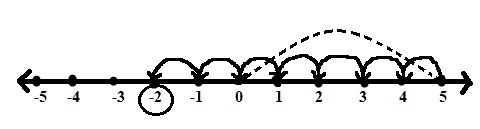(c) – 5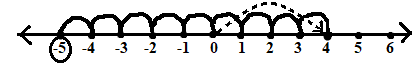(d) 1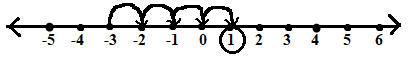(e) – 6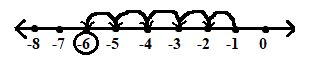(f) − 2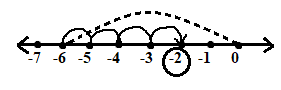8) Write the additive inverse of :
(a) – 27      (b) 3        (c) 0        (d) – 5
Ans:
(a) 27
(b) – 3
(c) 0
(d) 5

9) Write the successor and predecessor of :
(a) – 13        (b) 7           (c) −27
Ans:
(a) Successor :    −13 + 1 = − 12
Predecessor :    −13 – 1 = − 14
(b) Successor : 7 + 1 = 8
Predecessor : 7 – 1 = 6
(c) Successor :  − 27 + 1 = − 26
Predecessor :    − 27 −1 = −28

10) Joshua thinks of an integer. He subtracts 12 from it and gets the result                   as −6.What was the integer he thought of ?
Ans: Let the integer be x
Now, x – 12 = 6
x = 6 + 12
= 6
∴ The required integer is 6.